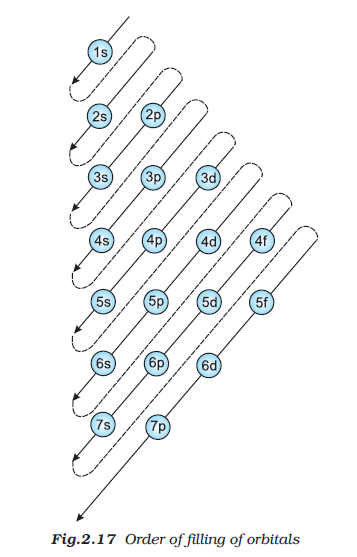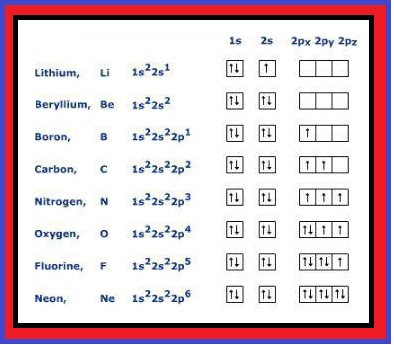Chemistry Filling of Atomic Orbitals and Electronic Configuration of Atoms
Click for Only Video

### Topics to be covered :

● Filling of Orbitals in Atom
● Aufbau Principle
● Pauli's Exclusion Principle
● Hund's Rule of Maximum Multiplicity
● Electronic Configuration of Atom
● Advantage of Orbital Diagram
● Importance of Electronic Configuration

### Filling of Orbitals in Atom :

There are three rules according to which electrons are filled into the orbitals of an atom.

### Aufbau Principle :Aufbau => means building up (in German )

Here, building up means => Filling up of orbitals with electrons.

Statement : In the ground state of the atoms, the orbitals are filled in order of the their increasing energies.

The order of filling of electrons into orbitals is as follows :

1s < 2s < 2p < 3s < 3p < 4 s < 3d < 4p < 5 s < 4d < 5p < ..............

Trick : See fig.2.17

### Pauli Exclusion Principle :

This principle was given by Walfgang Pauli (1926).

Statement : No two electrons in an atom can have the same set of four quantum numbers.

or Any two electrons can exist in the same orbital with opposite spin.

This rule helps in calculating the number of electrons a subshell can occupy.

So, the maximum number of electrons in a shell = 2n^2 and the maximum capacity of a sub-shell = 2(2l + 1)

### Hund's Rule of Maximum Multiplicity :

This rule is basically about the filling of electrons into the orbitals belonging to the same subshell.

Statement : Pairing of electrons in a orbital of a specific subshell (s, p, d, f) does not take place until each orbital of that subshell is singly occupied.

### Electronic Configuration of Atom :Definition : The distribution of electrons into orbitals of an atom is called its electronic configuration.

The electronic configuration of an atom is written according to the three principle studied above.

Electronic configuration of different atoms can be represented as :

(i) s^a , p^b , d^c ............. notation (a, b, c represent the no. of electrons in respective subshell)

(ii) orbital diagram (See Image)

In this, each box represent the orbital of a subshell.

The electron is represented by up arrow (↑) positive spin or a down arrow (↓) a negative spin.
Q 2642145933The electronic configuration of an element with atomic number 24 is

(A)1s^2 , 2s^2 , 2p^6 , 3s^2 , 3p^6 , 3d^4 , 4s^2

(B)1s^2, 2s^2, 2p^6, 3s^2, 3p^6, 3d^(10) ,

(C)1s^2, 2s^2, 2p^6, 3s^2, 3p^6, 3d^6,

(D)1s^2,2s^2,2p^6,3s^2,3p^6,3d^5,4s^1Solution:

The electronic configruation of element with atomic number 24 is

1s^2,2s^2,2p^6,3s^2,3p^6,3d^5,4s^1
Correct Answer is => (D) 1s^2,2s^2,2p^6,3s^2,3p^6,3d^5,4s^1
Q 2662145935What is the electronic configuration of Mn^(2+) ?

(A)[Ne] 3d^5 , 4s^0

(B)[Ar] 3d^5 , 4s^2

(C)[Ar] 3d^5 , 4s^0

(D)[Ne] 3d^5 , 4s^2Solution:

Electronic configuration of Mn (25) is

1s^2 , 2s^2 , 2p^6 , 3s^2 , 3p^6 , 4s^2 , 3d^5

therefore Electronic configuration of Mn^(2+) is

1s^2 , 2s^2 , 2p^6 , 3s^2 , 3p^6 , 3d^5

because Mn^(2+) = [Ar] 3d^5 , 4s^0
Correct Answer is => (C) [Ar] 3d^5 , 4s^0

### Advantage of Orbital Diagram :

It gives information about all the four quantum numbers.

Core electrons => These are electrons in the completely filled shells.

Valence electrons => These are the electrons in the shell with the highest principle quantum number.

### SPECIAL ELECTRONIC CONFIGURATION

=> In case of scandium, 3d orbital being lower in energy than 4p is filled first.
=> Now in scandium, titanium, vanadium, chromium, manganese, iron, cobalt, nickel, copper, zinc 3d orbitals are progressively filled.
Now comes the special discussion about half filled and fully filled configuration
=> Chromium and copper have five and ten d electrons instead of four and nine electrons in their 3d orbitals. This is because p^3, p^6, d^5, d^10, f^7, f^14 are either half filled or fully filled and are more stable.
=> With the saturation of 3d orbitals, the filling of 4p starts at gallium and is complete at krypton.
=> Now from rubidium to xenon the pattern of filling the 5s, 4d, 5p orbitals are similar to that of 4s, 3d, 4p orbitals.
=> In cesium and barium 6s conatins one and two electrons respectively.
=. Then from lanthanum to mercury filling of electrons takes place in 4f and 5d orbitals. After this filling of 6p, then 7s and finally 5f and 6d orbitals takes place.
=> The elements after uranium are all short lived and all of them are produced artificially.

### Importance of Electronic Configuration :

Electronic configuration is useful in understanding and explaining chemical behaviour.

Questions like => (a) why two or more atoms combine to form molecules?

(b) Why some elements are metals and some are non metals?

(c) Why He and Ar are not reactive but halogens are reactive?

Answer to all these questions are given based on electronic configuration of the element.